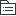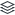# 奥数所有计算

|
4

## 相关模板推荐

•## 计算 —— 作品大纲

•整数
• 乘法分配律
• (a+b)c=a×c+b×c
• 设元法
• 注意：1.分组 2.设元（设小不设大） 3.注意变号。
• 平方差
• a的平方-b的平方=（a+b）×(a-b) 逆用：（a+b）(a-b)=a的平方-b的平方。
• 等差数列
• （首项+末项）×项数÷2。
• 平方和
• n×(n+1)×（2n+1）÷6
• 你×你哥×你俩一起出去溜
• 减法的性质
• a-b-c=a-（b+c）
• 除法的性质
• a÷b÷c=a÷（b×c）
• a÷b+a÷c=（a+b）÷c。
• 整数列项
• 1×2+2×3+3×4+4×5…+19×20=（19×20×21-0×1×2）÷3
• 前出后进大减小找准倍数要记牢。倍数怎么找连成个数成公差。
•分数计算
• 分数列项
• 分母积分子差
• 分母积分子不差
• 立方和
• 1³+2³+3³+……+n³=（1+2+3…n）²
• 姐妹花
• 先找姐妹花
• 拆大不拆小
• 整体约分
• 上下看，左右看。
• 分子是分母的倍数分母不变结果为倍数
• 分母是分子的倍数分子不变结果是倍数分之一
• 滚雪球
• 从小开始滚
• 大数×2-小数。